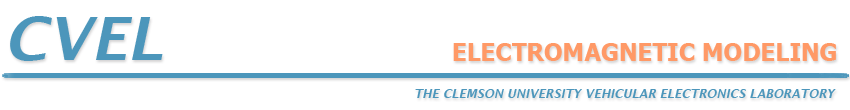### What is the "best" computational method?

The answer to this question depends to a great extent on the particular problem that is to be analyzed. Analytical methods are very good at analyzing certain problems with a high degree of symmetry and they can provide a great deal of insight into the behavior of many configurations. However, an accurate evaluation of most realistic electromagnetic configurations requires a numerical approach.

#### Method of Moments

Numerical techniques based on the method of weighted residuals are called moment methods. EM modelers have come to use the term "moment method" synonymously with "boundary element method". The boundary element method is a moment method applied to the solution of surface integral equations. Most commercial moment method codes are boundary element codes, however the method of weighted residuals can be applied to differential equations as well as integral equations. In general, moment method techniques do an excellent job of analyzing unbounded radiation problems and they excel at analyzing PEC (perfect electric conductor) configurations and homogeneous dielectrics. They are not well-suited to the analysis of complex inhomogeneous geometries.

#### Finite Element Method

Finite element techniques require the entire volume of the configuration to be meshed as opposed to surface integral techniques, which only require the surfaces to be meshed. However each mesh element may have completely different material properties from those of neighboring elements. In general, finite element techniques excel at modeling complex inhomogeneous configurations. However, they do not model unbounded radiation problems as effectively as moment method techniques.

#### Finite Difference Time Domain

Finite difference time domain (FDTD) techniques also require the entire volume to be meshed. Normally, this mesh must be uniform, so that the mesh density is determined by the smallest detail of the configuration. Unlike most finite element and moment method techniques, FDTD techniques work in the time domain. This makes them very well-suited to transient analysis problems. Like the finite element method, FDTD methods are very good at modeling complex inhomogeneous configurations. Also, many FDTD implementations do a better job of modeling unbounded problems than finite element modeling codes. As a result, FDTD techniques are often the method of choice for modeling unbounded complex inhomogeneous geometries.

#### Other Techniques

There are numerous other electromagnetic modeling techniques. Methods such as the Transmission Line Matrix Method (TLM), Generalized Multipole Technique (GMT), and others each have their own set of advantages for particular applications.

#### Reference

Survey of Current Computational Electromagnetics Techniques and Software
Clemson Vehicular Electronics Laboratory Technical Report: CVEL-08-011.2